### 測試(Testing)

```#![cfg(target_arch = "wasm32")]

extern crate wasm_bindgen_test;
use wasm_bindgen_test::*;

wasm_bindgen_test_configure!(run_in_browser);

#[wasm_bindgen_test]
fn pass() {
assert_eq!(1 + 1, 2);
}```

wasm-pack test --chrome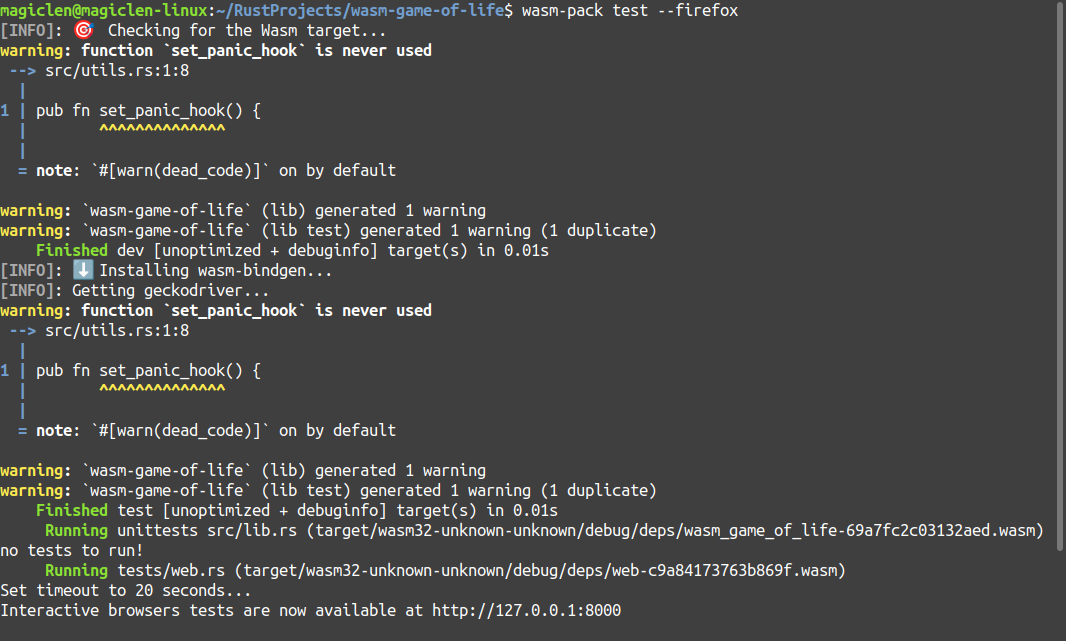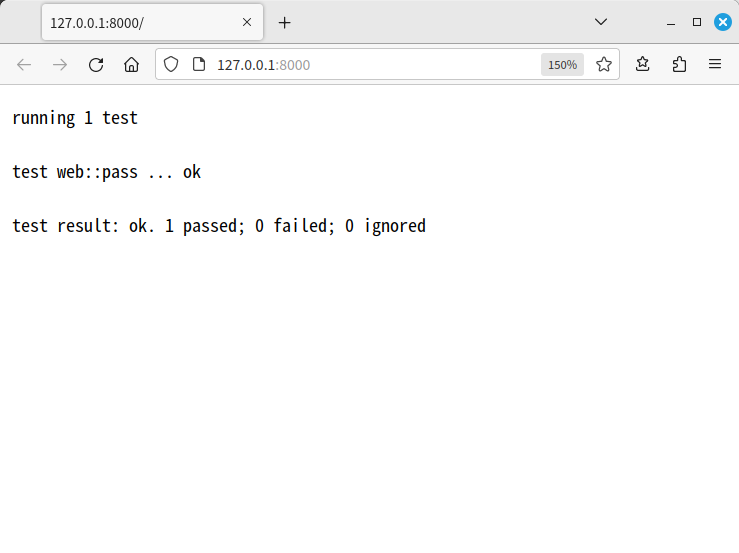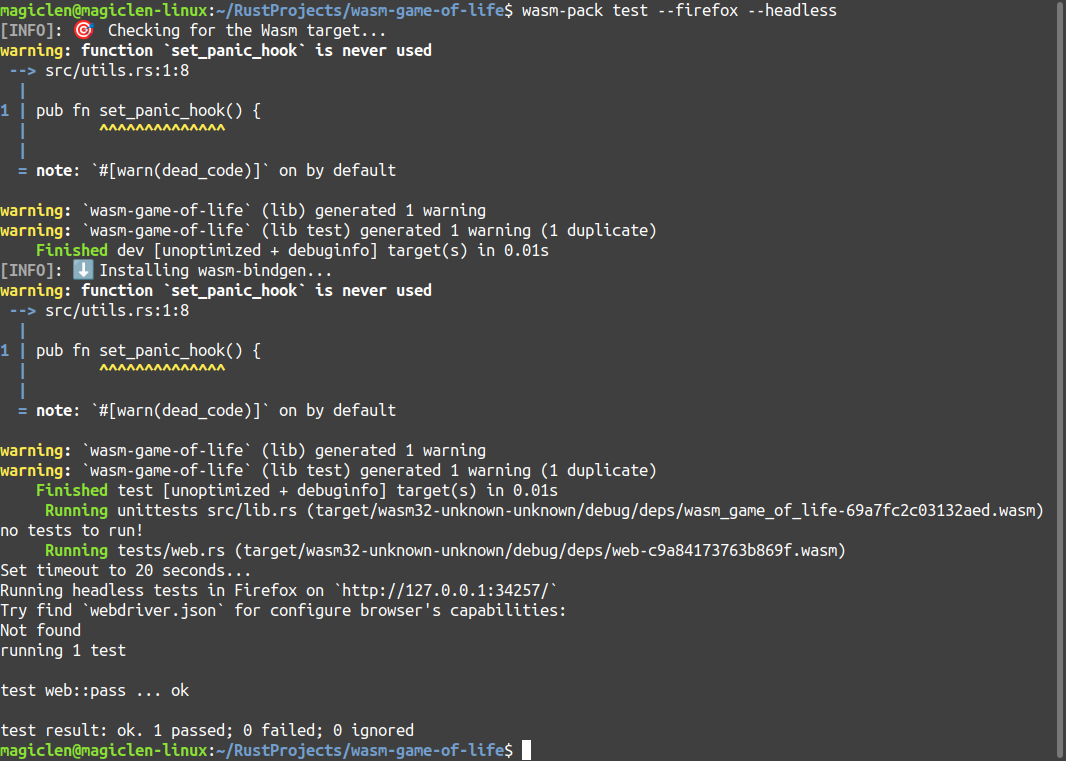#### 替康威生命遊戲撰寫WebAssembly測試

```impl Universe {
pub fn check_cells(&self, cells_indexes: &[usize]) -> bool {
for (index, block) in self.blocks.iter().enumerate() {
if cells_indexes.contains(&index) {
if let Block::NoCell = block {
return false;
}
} else if let Block::HasCell = block {
return false;
}
}

true
}

pub fn add_cells(&mut self, indexes: &[usize]) {
for &index in indexes {
self.blocks[index] = Block::HasCell;
}
}
}```

```#![cfg(target_arch = "wasm32")]

use wasm_bindgen_test::*;

use wasm_game_of_life::{Block, Universe};

wasm_bindgen_test_configure!(run_in_browser);

#[wasm_bindgen_test]
fn rule_1() {
let mut universe = Universe::new(5, 5);

universe.tick();

assert!(universe.check_cells(&[]));
}

#[wasm_bindgen_test]
fn rule_2() {
let mut universe = Universe::new(5, 5);

universe.tick();

assert!(universe.check_cells(&));
}

#[wasm_bindgen_test]
fn rule_3() {
let mut universe = Universe::new(5, 5);

universe.tick();

assert!(universe.check_cells(&[7, 8, 11, 13, 17]));
}

#[wasm_bindgen_test]
fn rule_4() {
let mut universe = Universe::new(5, 5);

universe.tick();

assert!(universe.check_cells(&));
}

#[wasm_bindgen_test]
fn case_1() {
let mut universe = Universe::new(5, 5);

universe.tick();

assert!(universe.check_cells(&[11, 12, 13]));
}```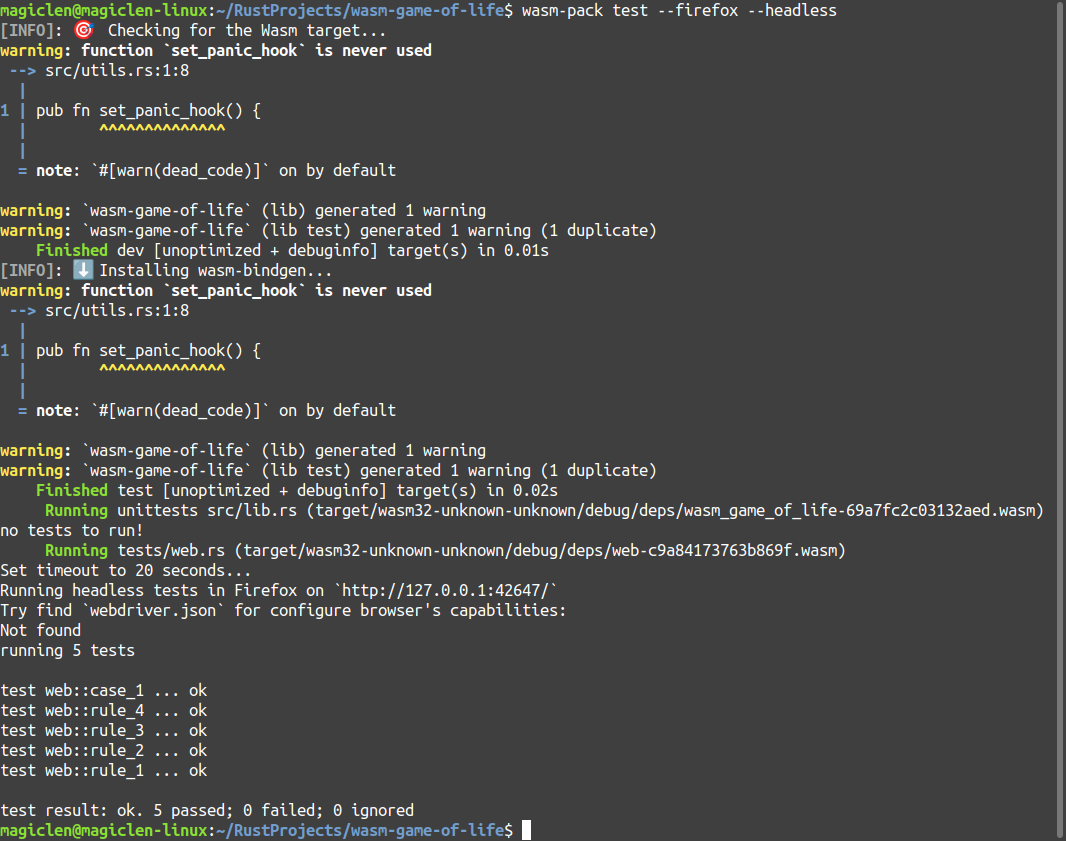### 偵錯(Debugging)

#### 顯示panic訊息

```#[wasm_bindgen]
impl Universe {
#[wasm_bindgen(constructor)]
pub fn new(width: u32, height: u32) -> Universe {
if cfg![debug_assertions] {
utils::set_panic_hook();
}

...
}

...
}```

#### 輸出訊息到主控台

```[dependencies.web-sys]
version = "0.3"
features = ["console"]```

```extern crate web_sys;

macro_rules! log {
( \$( \$t:tt )* ) => {
web_sys::console::log_1(&format!( \$( \$t )* ).into());
}
}```

`web_sys`這個crate提供的`log_1`函數即為JavaScript的`console.log`函數的只能傳一個參數的版本。`web_sys`也有提供能傳兩個參數的`log_2`函數，如果要傳三個參數就是用`log_3`，依此類推。不過由於這種API用起來有點麻煩，所以我們在這裡自訂了一個`log`巨集，就可以透過它來以類似`print!`巨集那樣的語法格式輸出訊息了！

`log!("A = {}, B = {}", 5, 6);`

A = 5, B = 6

```#[wasm_bindgen]
impl Universe {
pub fn tick(&mut self) {
...

for row in 0..self.height {
for column in 0..self.width {
...

let has_cell = self.has_cell(index);

let live_neighbor_cells_count = {
...
};

if cfg![debug_assertions] {
log!(
"Is blocks[{row:5}, {column:5}] has a live cell? {has_cell:5}. And it has {live_neighbor_cells_count} live neighbors.",
row = row, column = column, has_cell = has_cell, live_neighbor_cells_count = live_neighbor_cells_count
);
}

...
}
}

...
}
}```

#### 用JavaScript的`debugger`關鍵字建立偵錯點

```...

const renderLoop = () => {
debugger;

universe.tick();

drawCells();

requestAnimationFrame(renderLoop);
};

...```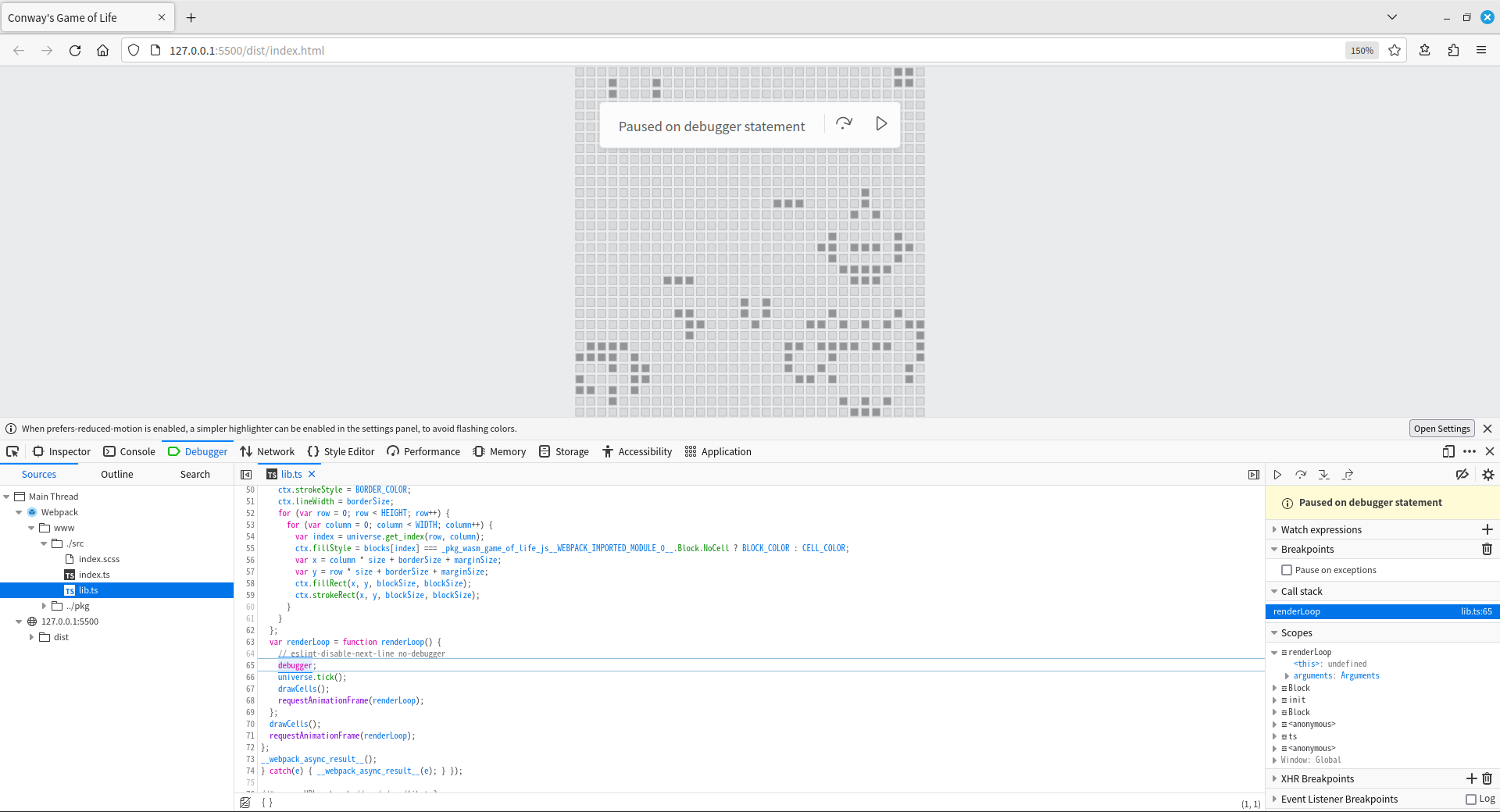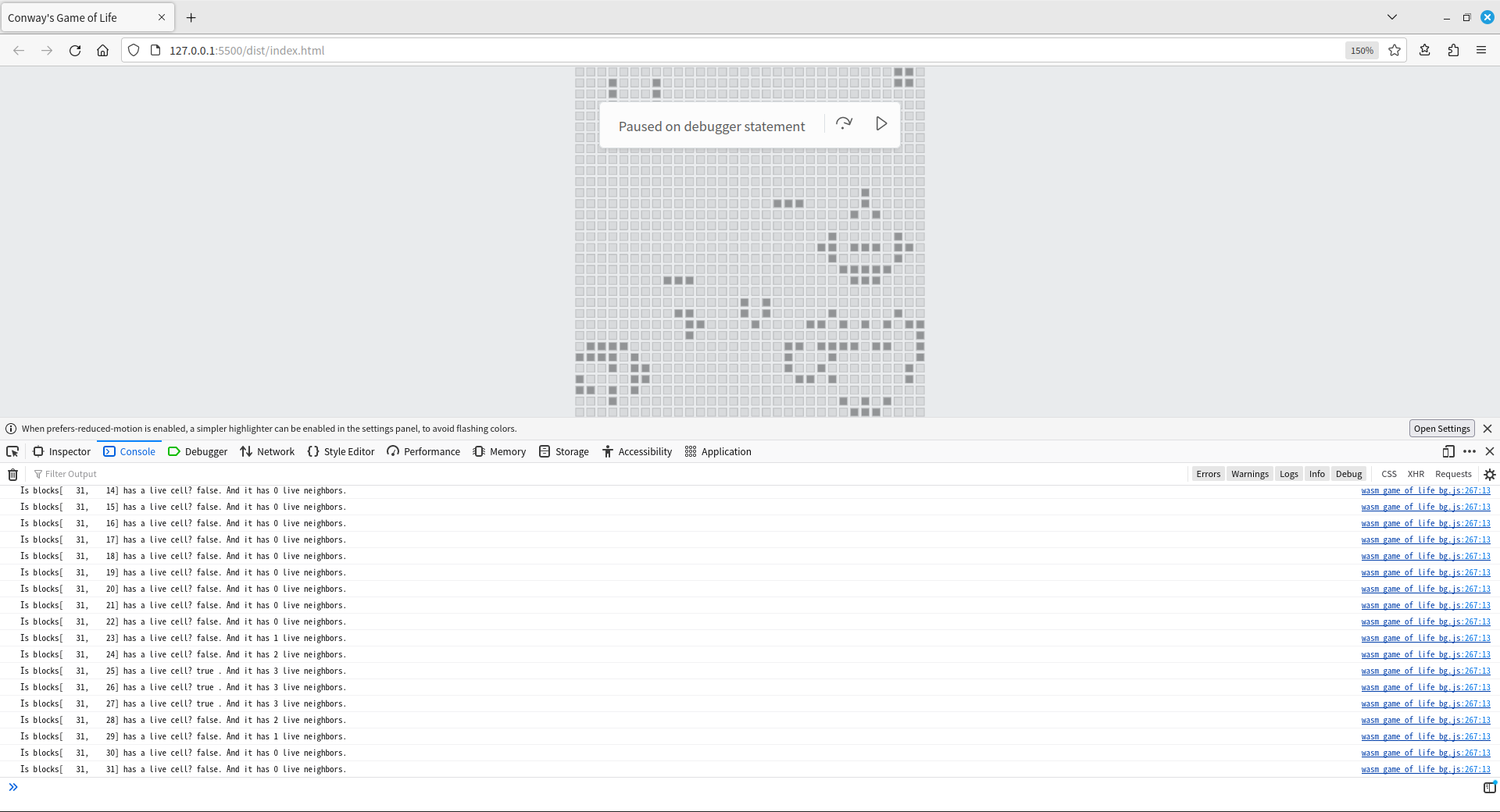#### 其它類型的訊息

```macro_rules! log {
( \$( \$t:tt )* ) => {
web_sys::console::log_1(&format!( \$( \$t )* ).into());
}
}

macro_rules! info {
( \$( \$t:tt )* ) => {
web_sys::console::info_1(&format!( \$( \$t )* ).into());
}
}

macro_rules! warn {
( \$( \$t:tt )* ) => {
web_sys::console::warn_1(&format!( \$( \$t )* ).into());
}
}

macro_rules! error {
( \$( \$t:tt )* ) => {
web_sys::console::error_1(&format!( \$( \$t )* ).into());
}
}```